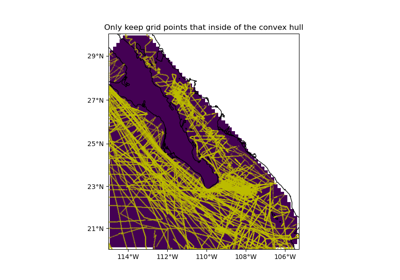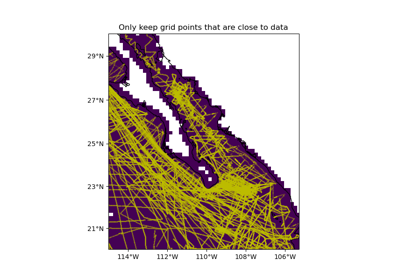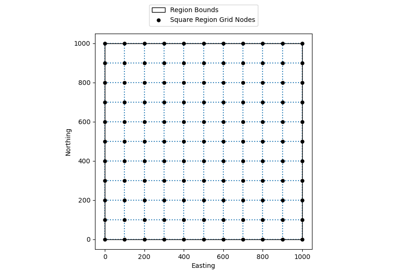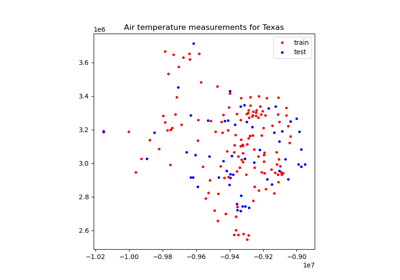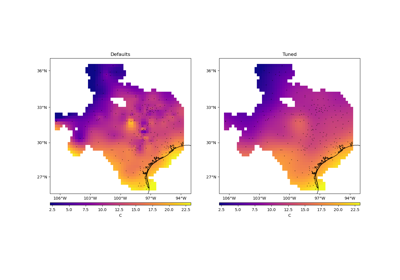# verde.grid_coordinates¶

verde.grid_coordinates(region, shape=None, spacing=None, adjust='spacing', pixel_register=False, extra_coords=None)[source]

Generate the coordinates for each point on a regular grid.

The grid can be specified by either the number of points in each dimension (the shape) or by the grid node spacing.

If the given region is not divisible by the desired spacing, either the region or the spacing will have to be adjusted. By default, the spacing will be rounded to the nearest multiple. Optionally, the East and North boundaries of the region can be adjusted to fit the exact spacing given. See the examples below.

Parameters
• region (list = [W, E, S, N]) – The boundaries of a given region in Cartesian or geographic coordinates.

• shape (tuple = (n_north, n_east) or None) – The number of points in the South-North and West-East directions, respectively.

• spacing (float, tuple = (s_north, s_east), or None) – The grid spacing in the South-North and West-East directions, respectively. A single value means that the spacing is equal in both directions.

• adjust ({'spacing', 'region'}) – Whether to adjust the spacing or the region if required. Ignored if shape is given instead of spacing. Defaults to adjusting the spacing.

• pixel_register (bool) – If True, the coordinates will refer to the center of each grid pixel instead of the grid lines. In practice, this means that there will be one less element per dimension of the grid when compared to grid line registered (only if given spacing and not shape). Default is False.

• extra_coords (None, scalar, or list) – If not None, then value(s) of extra coordinate arrays to be generated. These extra arrays will have the same shape as the others but will contain a constant value. Will generate an extra array per value given in extra_coords. Use this to generate arrays of constant heights or times, for example, that might be needed to evaluate a gridder.

Returns

coordinates (tuple of arrays) – Arrays with coordinates of each point in the grid. Each array contains values for a dimension in the order: easting, northing, vertical, and any extra dimensions given in extra_coords. All arrays will have the specified shape.

Examples

```>>> east, north = grid_coordinates(region=(0, 5, 0, 10), shape=(5, 3))
>>> print(east.shape, north.shape)
(5, 3) (5, 3)
>>> # Lower printing precision to shorten this example
>>> import numpy as np; np.set_printoptions(precision=1, suppress=True)
>>> print(east)
[[0.  2.5 5. ]
[0.  2.5 5. ]
[0.  2.5 5. ]
[0.  2.5 5. ]
[0.  2.5 5. ]]
>>> print(north)
[[ 0.   0.   0. ]
[ 2.5  2.5  2.5]
[ 5.   5.   5. ]
[ 7.5  7.5  7.5]
[10.  10.  10. ]]
```

The grid can also be specified using the spacing between points instead of the shape:

```>>> east, north = grid_coordinates(region=(0, 5, 0, 10), spacing=2.5)
>>> print(east.shape, north.shape)
(5, 3) (5, 3)
>>> print(east)
[[0.  2.5 5. ]
[0.  2.5 5. ]
[0.  2.5 5. ]
[0.  2.5 5. ]
[0.  2.5 5. ]]
>>> print(north)
[[ 0.   0.   0. ]
[ 2.5  2.5  2.5]
[ 5.   5.   5. ]
[ 7.5  7.5  7.5]
[10.  10.  10. ]]
```

The spacing can be different for northing and easting, respectively:

```>>> east, north = grid_coordinates(region=(-5, 1, 0, 10), spacing=(2.5, 1))
>>> print(east.shape, north.shape)
(5, 7) (5, 7)
>>> print(east)
[[-5. -4. -3. -2. -1.  0.  1.]
[-5. -4. -3. -2. -1.  0.  1.]
[-5. -4. -3. -2. -1.  0.  1.]
[-5. -4. -3. -2. -1.  0.  1.]
[-5. -4. -3. -2. -1.  0.  1.]]
>>> print(north)
[[ 0.   0.   0.   0.   0.   0.   0. ]
[ 2.5  2.5  2.5  2.5  2.5  2.5  2.5]
[ 5.   5.   5.   5.   5.   5.   5. ]
[ 7.5  7.5  7.5  7.5  7.5  7.5  7.5]
[10.  10.  10.  10.  10.  10.  10. ]]
```

If the region can’t be divided into the desired spacing, the spacing will be adjusted to conform to the region:

```>>> east, north = grid_coordinates(region=(-5, 0, 0, 5), spacing=2.6)
>>> print(east.shape, north.shape)
(3, 3) (3, 3)
>>> print(east)
[[-5.  -2.5  0. ]
[-5.  -2.5  0. ]
[-5.  -2.5  0. ]]
>>> print(north)
[[0.  0.  0. ]
[2.5 2.5 2.5]
[5.  5.  5. ]]
>>> east, north = grid_coordinates(region=(-5, 0, 0, 5), spacing=2.4)
>>> print(east.shape, north.shape)
(3, 3) (3, 3)
>>> print(east)
[[-5.  -2.5  0. ]
[-5.  -2.5  0. ]
[-5.  -2.5  0. ]]
>>> print(north)
[[0.  0.  0. ]
[2.5 2.5 2.5]
[5.  5.  5. ]]
```

You can choose to adjust the East and North boundaries of the region instead:

```>>> east, north = grid_coordinates(region=(-5, 0, 0, 5), spacing=2.6,
>>> print(east.shape, north.shape)
(3, 3) (3, 3)
>>> print(east)
[[-5.  -2.4  0.2]
[-5.  -2.4  0.2]
[-5.  -2.4  0.2]]
>>> print(north)
[[0.  0.  0. ]
[2.6 2.6 2.6]
[5.2 5.2 5.2]]
>>> east, north = grid_coordinates(region=(-5, 0, 0, 5), spacing=2.4,
>>> print(east.shape, north.shape)
(3, 3) (3, 3)
>>> print(east)
[[-5.  -2.6 -0.2]
[-5.  -2.6 -0.2]
[-5.  -2.6 -0.2]]
>>> print(north)
[[0.  0.  0. ]
[2.4 2.4 2.4]
[4.8 4.8 4.8]]
```

We can optionally generate coordinates for the center of each grid pixel instead of the corner (default):

```>>> east, north = grid_coordinates(region=(0, 5, 0, 10), spacing=2.5,
...                                pixel_register=True)
>>> # Raise the printing precision for this example
>>> np.set_printoptions(precision=2, suppress=True)
>>> # Notice that the shape is 1 less than when pixel_register=False
>>> print(east.shape, north.shape)
(4, 2) (4, 2)
>>> print(east)
[[1.25 3.75]
[1.25 3.75]
[1.25 3.75]
[1.25 3.75]]
>>> print(north)
[[1.25 1.25]
[3.75 3.75]
[6.25 6.25]
[8.75 8.75]]
>>> east, north = grid_coordinates(region=(0, 5, 0, 10), shape=(4, 2),
...                                pixel_register=True)
>>> print(east)
[[1.25 3.75]
[1.25 3.75]
[1.25 3.75]
[1.25 3.75]]
>>> print(north)
[[1.25 1.25]
[3.75 3.75]
[6.25 6.25]
[8.75 8.75]]
```

Generate arrays for other coordinates that have a constant value:

```>>> east, north, height = grid_coordinates(
...     region=(0, 5, 0, 10), spacing=2.5, extra_coords=57
... )
>>> print(east.shape, north.shape, height.shape)
(5, 3) (5, 3) (5, 3)
>>> print(height)
[[57. 57. 57.]
[57. 57. 57.]
[57. 57. 57.]
[57. 57. 57.]
[57. 57. 57.]]
>>> east, north, height, time = grid_coordinates(
...     region=(0, 5, 0, 10), spacing=2.5, extra_coords=[57, 0.1]
... )
>>> print(east.shape, north.shape, height.shape, time.shape)
(5, 3) (5, 3) (5, 3) (5, 3)
>>> print(height)
[[57. 57. 57.]
[57. 57. 57.]
[57. 57. 57.]
[57. 57. 57.]
[57. 57. 57.]]
>>> print(time)
[[0.1 0.1 0.1]
[0.1 0.1 0.1]
[0.1 0.1 0.1]
[0.1 0.1 0.1]
[0.1 0.1 0.1]]
```

`scatter_points`

Generate the coordinates for a random scatter of points

`profile_coordinates`

Coordinates for a profile between two points

## Examples using `verde.grid_coordinates`¶